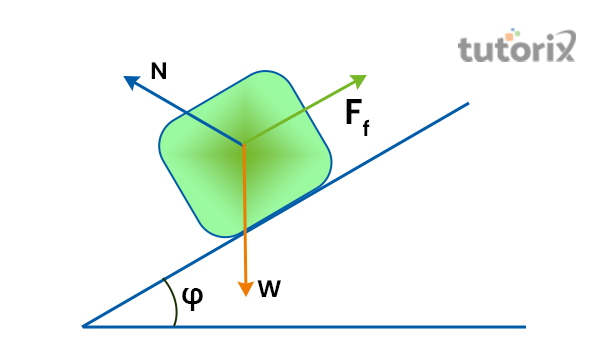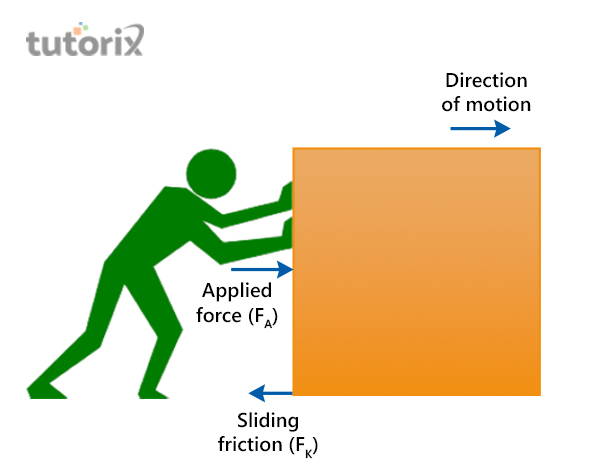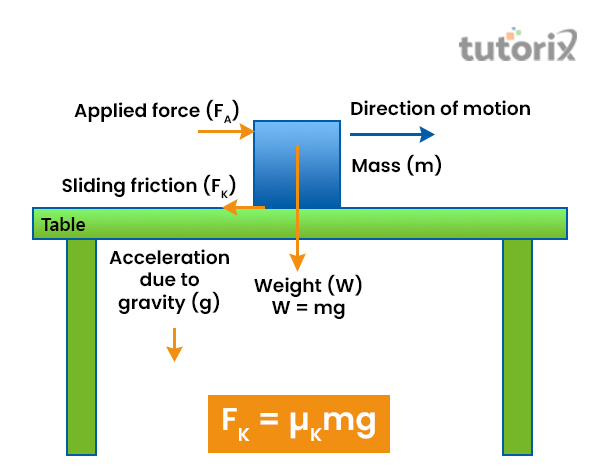# Sliding Friction

## Introduction

“Sliding friction” is an omnipresent force, in real-time daily operations. It is present in almost every form of human life and influences the motion of an object, especially when it is sliding on a surface or a floor. “Friction” is mostly generated when the floor or the surface is not perfect or smooth for an object to crawl. “Sliding friction” occurs when an object faces resistance while sliding over a bumpy floor in real-time.

## What is Sliding Friction?

“Sliding friction” can be defined as a “resistant force” that restricts the pathway of an object when it slides over a surface. When an object meets the floor, “sliding friction” is the force that creates obstacles for it to slide over the floor (Jia et al. 2022). For example, if a box is placed over a bumpy floor, it cannot slide easily over the floor because of the uneven force. Here, “sliding fraction” is creating resistance for the object to slide smoothly over the floor.Figure 1: Sliding Friction

“Sliding friction” is also known as “kinetic friction” due to its resistance over the pathway of an object. In simple words, “sliding friction” is a type of “kinetic friction” that is generated when “two surfaces” are coming into contact and is moving “relative to each other” (Sciencefacts, 2022). When “sliding friction” is applied to an object it is denoted with “FS = µSFn”. Here, the “FS” signifies the “force of the sliding action”, the “µS” means the “coefficient of sliding friction” and the “Fn” is the “normal force”. As a result, when a moving object is pushed over a bumpy surface, the “sliding friction” force appears as a resistant force that creates friction for the object to move smoothly on the floor.Figure 2: Examples of sliding friction

“Sliding friction” can also be defined as an “opposing force” that opposes the “movement between two objects”. This force is dependent on the “type of the contact surface”, especially the “finishing level of the floor” and the “materials used in making the surface” (Sciencefacts, 2022). For example, when a person slides a box over the floor, it makes direct contact with the floor sliding over it. Here, the person has to “overcome the resistance” of the floor in order to push the box over the floor successfully. Here, “sliding friction” appears as a “kinetic friction” that is restricting a person to push a box over the floor smoothly.

## Characteristics of Sliding Friction

“Sliding friction” is present almost everywhere, especially when force and resistance are generated with different actions in real-time. The force contains a few characteristics, especially when a force is applied to a moving object that is being slid over a surface. First, “sliding friction” is heavily dependent on the “contact force” it is facing while being applied to a moving object. Apart from that, “sliding friction” involves two different forces, the “applied force” and the “normal force” that is related to the moving object within an action (Jiang & Liu, 2022). Then, factors like the “direction of the motion” and the “mass of the object” affect the sliding of an object and the resistance over it.Figure 3: Characteristics of sliding friction

Characteristically, “sliding friction” is always “smaller than static friction” when it is applied to a moving object. It leads to unleashing another significant characteristic of “sliding friction”, the dependence of the force on the “nature of the material” (Sciencefacts, 2022). In addition, “sliding friction” appears as a “free force” in the “area of contact” when it is applied in real-time on an object (Elesa, 2022). It also opposes the “motion of an object” which makes it a resistant force, especially when an object is slid in the “opposite direction” with legitimate force.

## Factors affecting Sliding Friction in real-time

“Sliding friction” depends on multiple aspects related to the object, surface and the amount of applied force to an object. Initially, “sliding friction” is affected by the “deformation of objects”. For example, when components like air or water are placed against “solid surfaces” like rods, it characteristically depends on the “deformation of the road” (Geeksforgeeks, 2022). The “sliding friction” also depends on the “smoothness or the roughness” of the surface on which the object is being slid. In simple words, if the surface is smooth, the resistance from the surface is not going to be based on “sliding friction” (Geeksforgeeks, 2022). On the other hand, if an object is placed on a rough surface, it is going to experience more “sliding friction’’ as it is being heavily resisted by the unevenness of the floor.

## Conclusion

“Sliding friction” is a regular force that is applied when a moving object and a resistant surface make contact. If the floor has a smooth texture, the “sliding friction” appears smooth, but when the floor is rough, the “sliding friction” resists the movement of the object. In real-time, frictions are commonly generated by the unevenness of the surfaces where objects are being slid. In simple words, almost every motion where contact between a surface and an object is involved is an example of “siding friction”.

## FAQs

Q1. How the sliding friction can be reduced for an object?

Ans: “Sliding friction” can be reduced by “introducing lubricants” between the surface and the object. It can also be reduced by “using rollers” such as “ball bearing”, which follows “roller friction” force

Q2. Which factor can increase sliding friction?

Ans: Factors like “addition weight” can increase the “sliding friction” of an object. For example, if a person pushed two boxes at a time, the weight of the boxes can increase “sliding friction” for the objects.

Q3. What are some of the examples of sliding friction in real-time?

Ans: Real-time actions like “sledding”, “opening a window”, “sliding a car on ice”, and “skidding a car in a corner” are some of the examples of “sliding friction”.